# Numerical study of mixed convection and flow pattern in various across-shape concave enclosures

Numerical study of mixed convection and flow pattern in various across-shape concave enclosures

Laboratoire de Physique Théorique, Faculté de Technologie, Université de Bejaia, Bejaia 06000, Algérie

Laboratoire de Physique Théorique, Faculté des Sciences Exactes, Université de Bejaia, Bejaia 06000, Algérie

Laboratoire de Mécanique Matériaux et Énergétique, Faculté de Technologie, Université de Bejaia, Bejaia 06000, Algérie

Corresponding Author Email:
hamdimessaoud57@yahoo.com
Page:
567-575
|
DOI:
https://doi.org/10.18280/ijht.350313
|
Accepted:
|
Published:
30 September 2017
| Citation

OPEN ACCESS

Abstract:

Numerical study has been performed to investigate the combined effects of lid movement and buoyancy on flow and heat transfer characteristics for the mixed convective flow inside a lid-driven arc-shape cavity. The work is motivated by its immense importance due to its wide range of applications. The numerical simulations, therefore, are performed for three different shape concave enclosures (rectangular, circular and triangular) in laminar flow regime and for different Reynolds numbers (10 ≤ Re ≤ 1000) and Grashof numbers (104 ≤ Gr ≤ 107) effects on the flow and heat transfer. Numerical results are presented in terms of streamlines, isotherms, velocity profiles and average Nusselt number along the bottom wall. The comparisons showed that the increase of Reynolds and Grashof numbers enhance the heat transfer for all forms of alveolus. Further, triangular alveolus highlights a higher heat transfer rate for higher Re numbers.

Keywords:

mixed convection, driven cavity, shaped enclosure, finite volume method

1. Introduction

Combined convection flow occurs in lid-driven cavities due to both the shear force caused by the movement of the wall of the cavity and the buoyancy force produced by temperature difference on the boundary of the domain. Mixed convection problems in cavities are studied by several researchers in last two decades due to a range of applications. The combined convection flow and heat transfer in cavities find its applications in food processing and float glass production, solar collectors, lake and reservoirs, nuclear reactors, solar ponds and crystal growth  [1-5].

Many investigators studied the simple lid-driven rectangular and square cavities with temperature gradient (mixed convection) experimentally and numerically. A good review was reported by Basak et al.  which focused on the transition between natural and forced convection and between conduction and convection regimes. Ӧgüt  showed that the angle of inclination of the cavity affects the heat transfer and flow for large numbers of Ri. Ahmed et al.  have shown that the magnetic field and the inclination of the cavity greatly affects the heat transfer in a square driven cavity. Sun et al.  observed that the triangular fin is a good control parameter on heat transfer and flow field in a lid-driven square cavity. Zeghbib and Bessaïh  presented a study of laminar mixed convection in lid-driven cavities filled with Cu-water nanofluid heated by two heat sources placed on vertical walls.

Periodic wall heat flux is of great interest in engineering applications such as the design of cooling tubes for nuclear reactors or heat exchangers of Stirling engines, Zniper et al. . The sinusoidal wall heat flux is one of the simplest types of periodic heating and is encountered in many studies. Sarris et al.  investigated numerically the flow and heat transfer in a two-dimensional square cavity with a sinusoidal temperature profile at the upper wall and adiabatic conditions on the bottom and sidewalls. Pearlstein and Dempsey  studied a laminar tube flow subjected to axially varying wall heat flux in the form of a sinusoidal or hyperbolic tangent distribution. Barletta and Zanchini  studied the stationary and laminar forced convection in a circular tube with a sinusoidal axial distribution of wall heat flux, evaluating the heat transfer via the temperature fields and local Nusselt numbers.

In recent years many researchers have reported their research results about flow structure and heat transfer on a dimpled surface (non-rectangular geometry and on changes in the boundary conditions) such as Khanafer  studied numerically laminar mixed convection in lid driven cavity with a flexible heated bottom surface characterized by rectangular and sinusoidal wavy profiles. The investigation shows the benefits of using flexible walls when augmentation of heat transfer is sought at high Grashof numbers. Satyajit et al.  analyzed a T-shaped cavity filled with water-AL2O3 nanofluid, numerical solution is obtained for different solid volume fractions of nanofluid (φ=0-0.15), Grashof numbers (Gr = 0.1-5000) and Reynolds numbers (Re = 0.1-100). The result shows that all this parameters have significant effect on heat transfer characteristics inside the cavity. Chen and Cheng  investigate the steady laminar buoyancy-driven and convection heat transfer characteristics within three different across-shape concave enclosures. The effect of Prandtl number, Grashof number and the gap on the steady thermal behavior is examined. Results shows that the strength of the vortex is relatively bigger in the rectangular-rectangular concave enclosure than in the rectangular-circular concave enclosure at same Grashof number. Experimental and numerical study of flow over shallow cavities heated with constant heat flux from the bottom side has been carried by Mesalhy et al. . The effect of changing cavity aspect ratio (AR=L/H) and Reynolds number on flow field and heat transfer has been considered. They concluded that that the average Nusselt number increases as the cavity aspect ratio increase and heat transfer process depends strongly on Reynolds number for higthest aspect ratio. Experimental and numerical studies on periodic convection flow and heat transfer  in a lid-driven arc-shape cavity with temperature differential was investigated by Chen et al. . They studied three case corresponding to the Grashof numbers 2x105, 5x105 and 1.2x106 for a fixed Reynolds number (Re= 100). Results show that the periodic flow pattern occurs for the second Grashof number value and steady-state flow pattern take place in the other two cases. For periodic flow pattern, the central region in the cavity shows the larger oscillation of local kinetic energy and the longitudinal velocity component exhibits bigger oscillation amplitude in the upper region. Thus, the two colder regions in the upper part move from the left to the right with the right one gradually vanishing and the left one expanding as the lid moves rightward and continually. Recently Mliki et al.  studied laminar natural convection of Al2O3-H2O nanofluid in L-shaped cavity in the presence of a uniform magnetic field numerically by the Lattice Boltzmann Method.

The present study focuses on numerical simulations of mixed convection within a rectangular cavity with a shaped heated bottom wall (alveolus form). Special attention will be paid to the effects of the alveolus form (rectangular, circular and triangular) and Reynolds and Grashof numbers, on the flow field and heat transfer characteristics in the shaped cavity.

2. Problem Definition

The configuration problem is schematized in Fig. 1, corresponding to a rectangular cavity (height H1 and length L1) with presence of rectangular, triangular and circular alveolus (Length L2 and Height H2) on the bottom wall with aspect ratios of a = H1/L2 and b = L1/L2. The enclosure is filled with air (Pr = 0.71) and bounded by the heated differently surfaces such that hot bottom walls are at a temperature Th, the side walls are adiabatic whereas the top wall is assumed to slide from left to right with a constant velocity U0, is at a non-uniform temperature :

T = Tc+(Th-Tc) sin(px)/L.

The numerical model for mixed convection heat transfer in the shaped enclosure was developed under some assumptions as steady state, laminar and incompressible newtonian fluid. Viscous dissipation and compressibility effects are neglected. Also, the fluid properties are as sumed constant except the density in the buoyancy terms of the momentum equations, which can be approximated by the Boussinesq approach. Under these assumptions, the equations of continuity (1), momentum (2, 3) and  energy (4)  are cast in their dimensional form.

Continuity equation:

$\left(\frac{\partial U}{\partial X}+\frac{\partial V}{\partial Y}\right)=0$     (1)

Momentum equations:

$\left(U \frac{\partial U}{\partial X}+V \frac{\partial U}{\partial Y}\right)=-\frac{\partial P}{\partial X}+\frac{1}{\operatorname{Re}}\left(\frac{\partial^{2} U}{\partial X^{2}}+\frac{\partial^{2} U}{\partial Y^{2}}\right)$     (2)

$\left(U \frac{\partial V}{\partial X}+V \frac{\partial V}{\partial Y}\right)=-\frac{\partial P}{\partial Y}+\frac{1}{\operatorname{Re}}\left(\frac{\partial^{2} V}{\partial X^{2}}+\frac{\partial^{2} V}{\partial Y^{2}}\right)+\frac{G r}{\operatorname{Re}^{2}} \theta$      (3)

Energy equation:

$\left(U \frac{\partial \theta}{\partial X}+V \frac{\partial \theta}{\partial Y}\right)=\frac{1}{\operatorname{Pr} \operatorname{Re}}\left(\frac{\partial^{2} \theta}{\partial X^{2}}+\frac{\partial^{2} \theta}{\partial Y^{2}}\right)$     (4)

The boundary conditions, take the following form :

At the top wall

$\theta=\sin (\pi X)$ ,   U=1,  V=0      (5)

At the Bottom cavity and side walls of alveolus

q=1,  U=0, V=0      (6)

At the vertical side wall of the cavity

$\frac{\partial \theta}{\partial X}=0$ , U=0, V=0       (7)

In addition, the variables and dimensionless numbers are come follows:

X = x/L; Y = y/L; V = v/Uo;    U = u/Uo;

P = pL²/ $\rho$ ν²; q = (T-Tc)/ DT

The dimensionless groups appearing in the above equations are defined as follows :

$G r=g \beta\left(T_{h}-T_{c}\right) L^{3} / v^{2} ; \operatorname{Pr}=v / \alpha$

$\operatorname{Re}=U_{0} L / v ; R i=G r / \mathrm{Re}^{2}$

where u and v are the velocity along the axes x and y, p is the pressure, T is the temperature with Th the temperature and Tc the cold temperature. P, U, V and q are pressure, the horizontal and longitudinal velocity the dimensionless temperature respectively. Re, Gr, Ri and Pr represent the Reynolds, Grashof, Richardson and Prandtl numbers respectively.

The physical quantity of interest is the average Nusselt number, $\overline{N u}$ , which is calculated at the bottom wall by integration of local Nusselt number, Nu, over the wall as :

$N u_{X}=-\frac{\partial \theta}{\partial Y} ; \overline{N u}=\int_{0}^{L} N u_{X} d X$       (8)

## 1a.png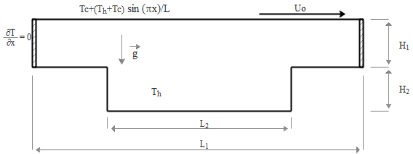a) Rectangular

## 1b.pngb) Triangular

## 1c.pngc) Circular

Figure 1. Schematic diagram of different shape concave, (alveolus)

3. Numerical Procedure

The numerical method used for discretizing the system of equations is the finite control volume method, Patankar . the combined continuity, momentum and energy equations (1)-(4) with the corresponding boundary conditions given in equations (5)-(7). The SIMPLER algorithm is utilized to solve the coupling between velocity and pressure and the convection-diffusion terms are discredited by a power-law scheme. The global criterion of convergence for the continuity, momentum an energy is set to 10-5 , 10-5 and 10-6 respectively.

3.1 Grid independency

A grid independency test has been carried out to find numerical accuracy while solving the system. the test is done for Gr=104 and Re=100. From Tab. 1, it is clear that the variation of the average Nusselt number is not influenced by the number of nodes from a 100x100 grid. Accordingly, a 120x120 uniform mesh is preferred keeping in view the accuracy of the results and the computation time.

3.2 Code validation

The present code is validated with the previous investigation by Ӧgüt  of square lid-driven cavity. Validation is established through streamlines, isotherms contours and average Nusselt number. Comparison of the flow and thermal field is illustrated in Fig. 2 and Tab. 2 for Ri=0.1, 1, 10 and 100. From the figure it is significant that the present code conforms to previous study and a good comparison is registered. According to this, the present numerical code and solution procedure are perfectly reliable. Hence, numerical simulation can be carried out for the given study.

Table 1. Grid independency

 Grid $\overline{N u}$ 60x60 2.288 80x80 2.299 100x100 2.303 120x120 2.308 140x140 2.310 160x160 2.311

Table 2. Comparison of average Nusselt with Ӧgüt 

 $\overline{N u}$ Ӧgüt  Present work Ri = 0.1 3.850 3.890 Ri = 1 2.602 2.631 Ri = 10 2.139 2.163 Ri = 100 1.884 1.906

## bu_huo_.pngFigure 2. Comparison of isotherms and streamlines for Ri=0.1

4. Results and Discussion

As mentioned earlier, the main objective of the present investigation is to compare flow and heat transfer characteristics between rectangular, circular and triangular alveolus on the bottom wall in lid-driven cavity. The transport phenomena are presented by analyzing streamlines, isotherms and average Nusselt number in the subsequent sections. In addition, the implications of varying Reynolds number, i e, Re = 10, 100, 500 and 1000 and Grashof number, i.e., Gr = 104, 105,106 and 107 will be investigated.

4.1 Flow and thermal fields

Figure 3 displays isotherms and streamlines for rectangular alveolus with Re = 10, for different Grashof numbers. Due to non-uniform heating, the temperature increases from the upper corners towards the bottom and top walls. And it is clearly observed that, the qualitative distributions of isotherms are almost invariant and symmetric for Gr ≤ 106, signifying that conduction is dominant heat transfer mechanism. As Gr increases temperature contour lines start getting deformed and lifted indicating that convective heat transfer is the dominant mode.

## 3.png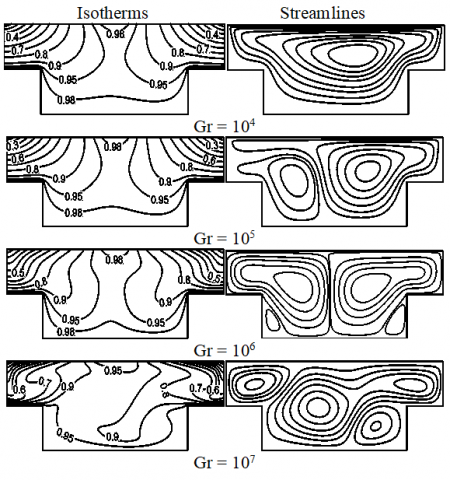Figure 3. Isotherms and streamlines for different Gr with rectangular case alveolus at Re = 10

However, the flow field is highly influenced by the motion of horizontal top wall as seen from the streamlines, note that a primary large clockwise circulation zone due to the lid movement along the positive x-direction, perfectly takes the shape of the cavity. At Gr = 105, a secondary anti-clockwise small vortex appears at the left side of the cavity, which increasing in size and the flow becames symmetric for Gr = 106. Increment of Gr to 107, generates two more secondary vortices inside the cavity, due to the intense effect of buoyancy force.

For Re = 1000, forced convection dominant flow occurs in all cases Fig. 4, The temperature contours are highly compressed and shifted on the right hand side of the enclosure. Furthermore, The streamline contours became two pairs of counter-rotating circulation cells, the primary vortex located in the upper part of the cavity and the secondary small vortex occupies the alveolus, which decreases gradually as the Grashof number increases.

## 4.pngFigure 4. Isotherms and streamlines for different Gr with rectangular alveolus case at Re = 1000

## 5.png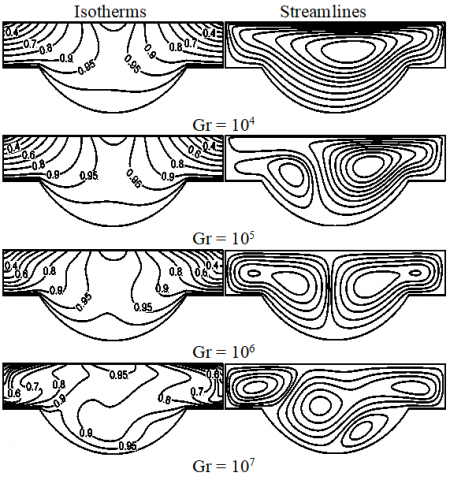Figure 5. Isotherms and streamlines for different Gr with circular alveolus case at Re = 10

Similar effects of Grashof and Reynolds numbers on the flow patterns and temperature distributions in the circular alveolus are shown in Figs. 5-6. It is observed that, at  Re=10 the isotherms are stratified and symmetrics for all Gr numbers except isotherms for  Gr = 107 which are curved an distorted on the middle of the enclosure. On the other hand, a large clockwise circulation fills almost the entire enclosure for Gr = 104. However, at Gr = 105, the streamline contours show two pairs of counter-rotating circulation eddies. As Grashof number increases to 106, the flow field becomes symmetric then multi-cellular for Gr = 107.

As Re increases to Re = 1000, enhances the effect of forced convection and suppresses the effect of natural convection as seen in Fig. 6. The isotherms profiles are not symmetric and are highly compressed and shifted on the right hand side of the cavity due to the increase of inertial force caused by the lid driven top wall.  Similar results for the flow pattern, a large clockwise circulation is induced by the motion of the top wall, occupies the major portion of the cavity and a secondary vortex in the alveolus, begins to grow slightly bigger with increasing of the Grashof number.

## 6.pngFigure 6. Isotherms and streamlines for different Gr with circular alveolus case at Re = 1000

## 7.png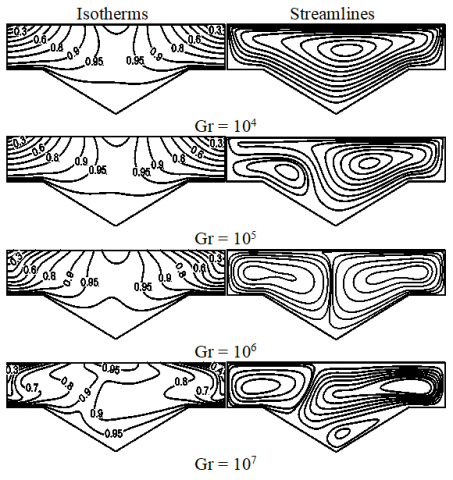Figure 7. Isotherms and streamlines for different Gr with triangular alveolus case at Re = 10

The predicted flow patterns and isotherms for triangular configuration are shown in Figs. 7-8 for different Gr and Re numbers. For small values of Re (10) and Gr (104), the fluid motion involves a primary recirculating eddie of relatively weak velocity extending throughout the cavity with clockwise direction. With the increase of Gr, due to the important temperature gradient the convection increases significantly and becomes stronger, such that a multi-cell structure prevails in the enclosure for Gr = 106. The increase of buoyancy effect tend to produce a minor vortices in the top left side and in bottom part of the cavity  for Gr=107. The profiles of isotherms are symmetric with respect to vertical center line and are very similar to those rectangular and circular profiles.

## 8.pngFigure 8. Isotherms and streamlines for different Gr with triangular alveolus case at Re = 1000

For largest values of Re (1000), forced convection dominates, Fig. 8. A large clockwise circulation fills almost the entire enclosure due to the sliding wall, while the strength of the secondary vortex located in the  alveolus increases with increasing Gr. The dominance of lid velocity is pronounced in the isotherms contours in the all cases, the isotherms are smooth and continuous curves throughout the entire cavity and are shifting from the right hand side of the cavity.

4.2 Profiles of the velocity

Figure 9 illustrates the evolutions of horizontal velocity in the middle of the cavity X = 0.5, for different shape of  alveolus and for Re = 10, 1000 and Gr = 104, 107. It indicates that the velocity shows that the profile for Re = 10, Gr = 104 has one turning point, implying that the flow field consists of unicellular pattern. The profiles for Re = 1000 and Gr = 104 have two points of inflection indicating that the flow pattern is characterized by two-cell vortices. Their distribution remains almost similar in shape observed for all kinds of alveolus. In addition, it's noted that the velocity profile of rectangular alveolus is higher than the other forms of alveolus.

On the other hand, velocity profile has a slower variation in the core region and a larger gradient closer to the moving lids. For high Reynolds number and small Grashof number (Re = 1000 and Gr = 104), the dominance of the inertial forces can be noticed, which leads to a larger gradient close to the walls and a criss-cross variation in the core region. As Gr increases to 107, the profiles became symmetric signifying that the convection leads the flow inside the enclosure.

## 9a.png## 9b.png## 9c.png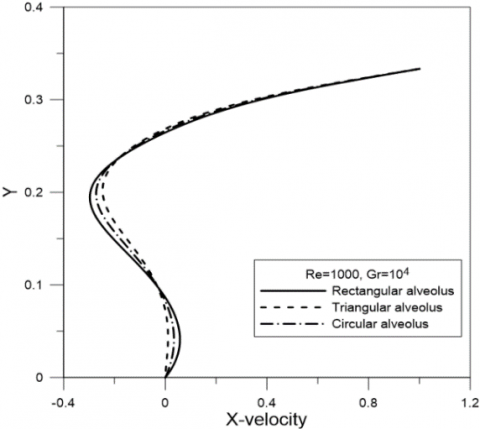## 9d.png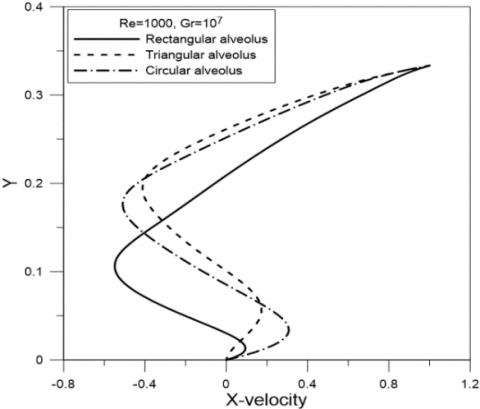Figure 9. The horizontal X-velocity profiles at the vertical mid-plane, for different shape of alveolus

4.3 Heat transfer

Average Nusselt Number, as a function of Gr number for different Re numbers, is analyzed in Figs. 10-11 for rectangular, circular and triangular alveolus respectively.

As can be seen from the Figure 10, that the mean Nusselt remain constant, which means that heat transfer mechanism is by pure conduction for Grashof number Gr < 105. However, it may be noted that all convection dominant states corresponding to Gr ≥ 105 show significantly higher heat transfer rate whatever the shape of alveolus. In addition, the average Nusselt number increases with the increase of Reynolds number, in accordance with previous observations.

It is noticed from Figure 11, that the shape of alveolus does not entail any significant change on the heat transfer for low Re (Re = 10) and Nu remains unchanged whether increasing Gr. Also, for greater Re (Re=103), triangular alveolus shows higher heat transfer rate followed by circular and rectangular shape, respectively. However, when Gr= 107 rectangular alveolus shows the higher heat transfer rate.

## 10a.png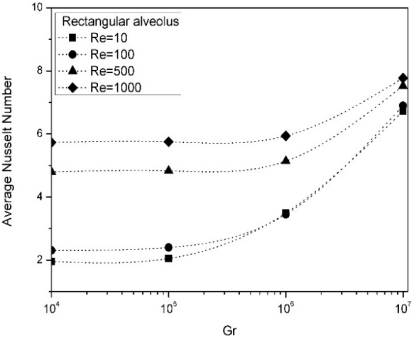a)

## 10b.pngb)

## 10c.png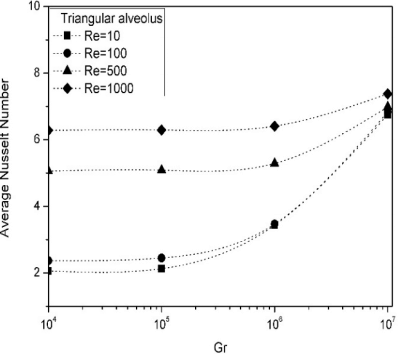c)

Figure 10. Variation of the average Nusselt number versus Gr with different Re, for a) rectangular, b) circular and c) triangular alveolus

## 11a.png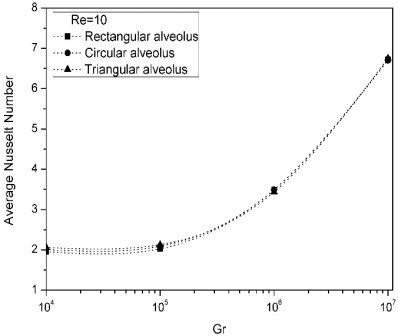a)

## 11b.png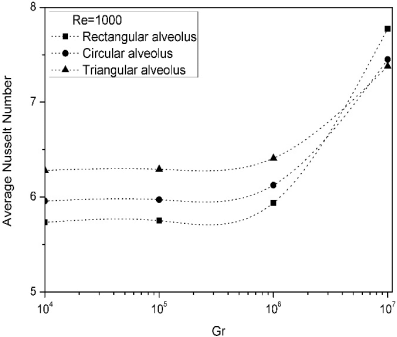b)

Figure 11. variation of the average Nusselt number versus Gr for a) Re = 10, b) Re = 1000

5. Conclusion

A volume finite  method is used to investigate the combined effect of moving lid and imposed buoyancy opposed temperature gradients on flow field and temperature distribution inside a rectangular enclosure with different form of alveolus (micro-cavity) such as ; rectangular, triangular and circular on the hot bottom wall. In each case, interplay between forced convection due to moving lid and natural convection due to temperature gradients are analyzed by varying Grashof (Gr) and Reynolds (Re) numbers. The following conclusions have been observed during the present study.

1.The natural convection parameter Gr and forced convection parameter Re effect significantly the flow and thermal current activities. Firstly the increase of Grashof number tends to make the flow two counter- clockwise circulations and increase the lifting and deformation of isotherms (convection dominance transfer). On the other hand the increase of Reynolds number tends to make the flow bi-cellular, a large  vortex occupies the cavity and small vortex occupies the alveolus, and the isotherms are shifted on the right hand side of the cavity (forced convection dominance).

2. The heat transfer is an increasing function of the Reynolds and Grashof numbers for all shape of alveolus.

3. For small Re numbers, the form of alveolus has no effect on the heat transfer.

4. For high Re numbers, triangular alveolus shows the higher heat transfer rate.

In summary, concave enclosure may play an important role in the flow and heat transfer characteristics. Further, this study suggest several guidelines for thermal design in engineering process and emphasizes the interest of the alveolus shape for a better thermal efficiency.

Nomenclature
 a, b aspect ratio a = H1/L2 , b = L1/L2 CP specific heat , J. kg-1. K-1 H1, H2 height of the cavity and the alveolus, m L1, L2 width of the cavity and the alveolus, m g gravitational acceleration, m.s-2 Gr Grashof number Nu Nusselt number k thermal conductivity, W.m-1. K-1 p Pressure, Pa P dimensionless pressure Pr Prandtl number Re Reynolds number Ri Richardson number T Temperature , K u, v velocity components in x, y directions, m.s-1 U,V dimensionless velocity components U0 velocity of the top wall, m.s-1 x, y Cartesians coordinates, m X, Y dimensionless coordinates Greek Symbols a thermal diffusivity, m2. s-1 b thermal expansion coefficient, K-1 θ dimensionless temperature ν kinematic viscosity, m2. s-1 Subscripts h hot temperature c cold temperature
References

 Pilkington L.A.B. (1969). Review lecture: The float glass process, Proc. Roy. Soc. London, Vol. 314, No, 1516, pp. 1-25. DOI: 10.1098/rspa.1969.0212

 Imberger J., Hamblin P.F. (1982). Dynamics of lakes, reservoirs, and cooling ponds, Annual Rev. Fluid Mech. Vol. 14, pp. 153-187. DOI: 10.1146/annurev.fl.14.010182.001101

 Cha C.K., Jaluria Y. (1984). Recirculating mixed convection flow for energy extraction, Int. J. Heat Mass transfer, Vol. 27, pp. 1801-1810. DOI:10.1016/0017-9310(84)90162-5

 Aissaoui F., Benmachiche A.H., Brima A., Bahloul D., Belloufi Y. (2016). Experimental and theoretical analysis on thermal performance of the flat plate solar air collector, International Journal of Heat and Technology, Vol. 34, No. 2, pp. 213-220. DOI: 10.18280/ijht.340209

 Kaliakatsos D., Cucumo M., Ferraro V., Mele M., Galloro A., Accorinti F. (2016). CFD analysis of a pipe equipped with twisted tape, International Journal of Heat and Technology, Vol. 34, No. 2, pp. 172-180. DOI: 10.18280/ijht.340203

 Basak T., Roy S., Sharma P.K., Pop I. (2009). Analysis of mixed convection flows within a square cavity with uniform and non-uniform heating of bottom wall, Inter. J. Thermal Sciences, Vol. 48, pp. 891-912. DOI: 10.1016/j.ijthermalsci.2008.08.003

 Ӧgüt E.B. (2010). Mixed convection in an inclined lid- driven enclosure with a constant flux heater using differential quadrature (dq) method, International Journal of the Physical Sciences, Vol. 5, No. 15, pp. 2287-2303.

 Ahmed S.E., Mansour M.A., Mahdy A. (2013). MHD mixed convection in an inclined lid-driven cavity with opposing thermal buoyancy force: Effect of non-uniform heating on both sidewalls, Nuclear Engineering and Design, Vol. 256, pp. 938-948. DOI: 10.1016/j.nucengdes.2013.06.023

 Sun C., Yu B., Oztop H.F., Wang Y., Wei J. (2011). Control of mixed convection in lid-driven enclosures using conductive triangular fins, International Journal of Heat and Mass Transfer, Vol. 54, pp. 894-909. DOI: 10.1016/j.ijheatmasstransfer.2010.09.068

 Zeghbid I., Bessaïh R. (2015). Mixed convection in lid-driven cavities filled with a nanofluid, International Journal of Heat and Technology, Vol. 33, No. 4, pp. 77-84. DOI: 10.18280/ijht.330410

 Zniper K., Oubarta A., Lahjomri J. (2005). Analytical solution to the problem of heat transfer in an MHD flow inside a channel with prescribed sinusoidal wall heat flux, Energy Convers. Manage. Vol. 46, No. 7-8, pp. 1147-1163. DOI: 10.1016/j.enconman.2004.06.023

 Sarris I.E., Lekakis I., Vlachos N.S. (2002). Natural convection in a 2D enclosure with sinusoidal upper wall temperature, Numerical Heat Transfer, Part A, Vol. 42, pp. 513-530. DOI: 10.1080/10407780290059675

 Pearlstein A.J., Dempsey B.P. (2001). Low Peclet number heat transfer in a laminar tube flow subjected to axially varying wall heat flux, ASME Journal of Heat Transfer, Vol. 110, No. 3, pp. 796-798. DOI: 10.1115/1.3250565

 Barletta A., Zanchini E., (1995). Laminar forced convection with sinusoidal wall heat flux distribution: Axially periodic regime, Heat Mass Transfer. Vol. 31, No. 1-2, pp. 41-48. DOI: 10.1007/BF02537420

 Khanafer K. (2014). Comparison of flow and heat transfer characteristics in a lid-driven cavity between flexible and modified geometry of a heated bottom wall, International Journal of Heat and Mass Transfer, Vol. 78, pp. 1032-1041. DOI: 10.1016/j.ijheatmasstransfer.2014.07.06

 Satyajit M., Sourav S., Sumon S., Mamun, M.A.H. (2015). Combined effect of Reynolds and Grashof numbers on mixed convection in a lid-driven T-shaped cavity filled with water-Al2O3 nanofluid, Journal of Hydrodynamics. Vol. 27, No. 5, pp. 782-794. DOI: 10.1016/S1001-6058(15)60540-6

 Chen C.L., Cheng C.H. (2011). Predictions of buoyancy – induced flow in various across-shape concave enclosures, International Communications in Heat and Mass Transfer, Vol. 38, pp. 442-448. DOI: 10.1016/j.icheatmasstransfer.2010.12.027

 Mesalhy O.M., Abdel Aziz S.S., El-Sayed M.M. (2010). Flow and heat transfer over shallow cavities, International Journal of Thermal Sciences, Vol. 49, pp. 514-521. DOI: 10.1016/j.ijthermalsci.2009.09.007

 Chen C.L., Chung Y.C., Lee T.F. (2012). Experimental and numerical studies on periodic convection flow and heat transfer in a lid-driven arc-shape cavity, International Communications in Heat and Mass Transfer, Vol. 39, pp. 1563-1571. DOI: 10.1016/j.icheatmasstransfer.2012.10.003

 Mliki B., Abbassi M.A., Omri A. (2016). Lattice Boltzmann simulation of magnet hydrodynamics natural convection in an L-shaped enclosure, International Journal of Heat and Technology, Vol. 34, No. 4, pp. 565-573. DOI: 10.18280/ijht.340403

 Patankar S.V. (1980). Numerical heat transfer and fluid flow, Hemisphere Publishing Corporation, New York.